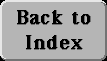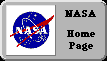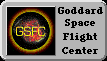# (21a) Applying Kepler's Third Law

For circular orbits around Earth, we found

T2 = (4p2/g RE2) r3

with T in seconds and r in meters. The distance of a satellite from the center of Earth in meters is an inconveniently big number, even before we raise it to the 3rd power. We may however multiply the expression on the right by (RE3/RE3) = 1 and then rearrange the terms:

T2 = (4p2/g RE2) (RE3/RE3) r3 = (4p2RE/g) (r/RE)3

The ratio r' = (r/RE) is the orbital distance measured in units of the Earth's radius. That number is usually between 1 (at the Earth's surface, r = RE) and 60 (at the Moon's orbit, r @ 60 RE).

Also, that ratio is always the same, whether r and RE are in meters, yards or nautical miles, as long as both r and RE are measured in the same units. The other term is calculated below, with multiplication denoted by blank spaces between parentheses; you may check it with your calculator.

(4p2RE/g) = (4) (9.87) (6 371 000)/9.81 = 25 638 838

Using computer notation for the square root

SQRT (25 638 838) = 5063.5 From this

T2= (5063.5)2 (r')3

T= 5063.5 seconds SQRT(r')3 = 5063 sec r'SQRT=(r')

This is the practical form of Kepler's 3rd law for Earth satellites. Our imagined satellite skimming the surface of the Earth (r' = 1) has a period

T = 5063.5 sec = (5063.5/60) minutes = 84.4 minutes

The space shuttle must clear the atmosphere and goes a bit higher. Say it orbits at r' = 1. 05, with SQRT(r') = 1. 0247. Then

T = (5063.5) (1.05) (1.0247) = 5448 seconds = 90.8 minutes

International communication satellites are in the equatorial plane of the Earth and have orbits with a 24-hour period. As the Earth's rotates, they keep pace with it and always stay above the same spot. What is their distance?

Here T is known and we need to find r':

T = 24 hours = 86 400 sec = 5063.5 SQRT(r')3

SQRT(r')3 = 86 400/5063.5 = 17.0632

If all numbers on the last line are equal, their squares are equal, too

(r')3 = (17.0632)2 = 291.156

Now you need a calculator able to derive cubic roots (or else, the 1/3 = 0.333. . . power). This gives